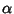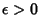## Roth's Theorem

For Algebraicwith, has finitely many solutions. Klaus Roth received a Fields Medal for this result.

See also Hurwitz Equation, Hurwitz's Irrational Number Theorem, Lagrange Number (Rational Approximation), Liouville's Rational Approximation Theorem, Liouville-Roth Constant, Markov Number, Segre's Theorem, Thue-Siegel-Roth Theorem

References

Davenport, H. and Roth, K. F. Rational Approximations to Algebraic Numbers.'' Mathematika 2, 160-167, 1955.

Roth, K. F. Rational Approximations to Algebraic Numbers.'' Mathematika 2, 1-20, 1955.

Roth, K. F. Corrigendum to `Rational Approximations to Algebraic Numbers'.'' Mathematika 2, 168, 1955.